# Oberseminar Analysis Winter 2020/2021

## Organizers: S. Conti, H. Koch, S. Müller, B. Niethammer, M. Rumpf, C. Thiele, J.J.L. Velázquez, T. Pernas-Castano

• Thursday, Nov 19 at 2:15 pm, Zoom meeting (Meeting ID: 991 3632 7869, Passcode: 425173)
Sebastian Hensel (Institute of Science and Technology Austria)
Uniqueness properties of weak solution concepts for curvature driven interface evolution in geometry and fluid mechanics.
Due to the occurrence of topology changes and geometric singularities, the existence theory for interface evolution problems typically relies on weak solution concepts. In the absence of a comparison principle, the question of their uniqueness properties however remained essentially unexplored for a long time. In this talk, I will present weak-strong uniqueness principles for two important interface evolution problems not admitting a comparison principle: i) the flow of two incompressible, viscous and immiscible fluids in the presence of surface tension, and ii) multiphase mean curvature flow. More precisely, for these models we establish uniqueness of a suitable class of weak solutions within the class of classical solutions prior to the first topology change. The key ingredient to these qualitative uniqueness results are quantitative stability estimates in terms of a novel notion of relative entropies for interface evolution problems. I will explain this concept first in a simple two-phase setting. In a second step, I discuss how the corresponding multiphase analogue leads to a gradient flow generalization of a well-known concept from minimal surface theory: the notion of calibrations. I conclude with an outlook on possible future directions. The talk is based on joint works with Julian Fischer, Tim Laux and Theresa Simon.

• Thursday, Nov 26 at 2:15 pm, Zoom meeting (Meeting ID: 991 3632 7869, Passcode: 425173)
Shengwen Wang (Queen Mary University of London)
A Brakke type regularity for the Allen-Cahn flow.
We will talk about an analogue of the Brakke's local regularity theorem for the $\epsilon$ parabolic Allen-Cahn equation. In particular, we show uniform $C_{2,\alpha}$ regularity for the transition layers converging to smooth mean curvature flows as $\epsilon$ tend to 0 under the almost unit-density assumption. This can be viewed as a diffused version of the Brakke regularity for the limit mean curvature flow. This talk is based on joint work with Huy Nguyen.

• Thursday, Dec 3 at 2:15 pm, Zoom meeting (Meeting ID: 991 3632 7869, Passcode: 425173)
Juán Carlos Felipe Navarro (BGSMath-UPC)
The Neumann problem for the fractional Laplacian: boundary regularity.
This talk will be devoted to introduce the Neumann problem for the fractional Laplacian, in contrast with the Dirichlet problem, focusing on recent results concerning the boundary regularity. First, we are motivating the problem through a probabilistic interpretation. Next, we are giving basic properties of the problem. Finally, we are presenting our recent boundary regularity results, as well as the main ideas appearing in the proofs. This is a joint work with Alessandro Audrito (ETH-Zurich) and Xavier Ros-Oton (ICREA-UB).

• Thursday, Dec 10 at 2:15 pm, Zoom meeting (Meeting ID: 991 3632 7869, Passcode: 425173)
Leon Bungert (FAU Erlangen-Nürnberg)
Asymptotic Behavior of Gradient Flows and Nonlinear Spectral Theory.
In this talk I will discuss the gradient flow of absolutely p-homogeneous convex functionals on a Hilbert space and show that asymptotic profiles of the solution are eigenfunctions of the subdifferential operator of the functional. This work applies, for instance, to local and nonlocal versions of PDEs like p-Laplacian evolution equations, the porous medium equation, and fast diffusion equations, herewith generalizing many results from the literature to an abstract setting. Then I discuss the eigenvalue problem associated to a infinity-Dirichlet energy in some more detail and show relations to distance functions. I conclude with extensions of the theory to metric gradient flows and future work.

• Thursday, Dec 17 at 2:15 pm, Zoom meeting (Meeting ID: 991 3632 7869, Passcode: 425173)
Kunlun Qi (City University of Hong Kong)
On the measure valued solution to the Boltzmann Equation with relevant numerical method.
In this talk, the well-posed theory of the measure valued solution to the Boltzmann equation and related model will be discussed, where a new geometric relation of both elastic and inelastic collision mechanism is applied to handle the strong singularity of the non-cutoff collision kernel. What’s more, a self-similar profile is proved to be the large-time asymptotic solution with the help of the stability analysis in a certain sense. At last, the computational approximation of the Boltzmann collision operator by our newly-proposed fast spectral method will be presented along with some numerical results. The talk is based on joint works with Tong Yang (CityU) and Jingwei Hu (Purdue).

• Thursday, Jan 14 at 2:15 pm, Zoom meeting (Meeting ID: 991 3632 7869, Passcode: 425173)
Tim Jahn (Institut für Mathematik, Goethe-Universität Frankfurt am Main)
Regularising linear inverse problems under unknown non-Gaussian noise.
We deal with the solution of linear ill-posed equations in Hilbert spaces. Usually, ne only has a corrupted measurement of the right hand side at hand and the Bakushinskii veto tells us, that we are not able to solve the equation if we do not know the noise level. But in applications such ad hoc knowledge may often be unrealistic. However, the error of a measurement may often be estimated through averaging of repeated measurements. We integrated that in our analysis and obtained convergence to the true solution, with the only assumption that the measurements are unbiased, independent and identically distributed according to an elseways arbitrary unknown distribution (with finite variance or of white noise type).

• Thursday, Jan 21 at 2:15 pm, Zoom meeting (Meeting ID: 991 3632 7869, Passcode: 425173)
Jeremy Michael Budd (Technische Universiteit Delft)
Theory and Image Segmentation with Graph MBO and Allen--Cahn.
Graph-based learning is an emerging technique for solving learning problems, e.g. segmentation and classification, by expressing these problems in the language of graph theory. One strand of this employs the dynamics of flows defined on a graph in order to classify its vertices. In particular, Bertozzi and co-authors considered dynamics related to Allen-Cahn flow (Bertozzi, Flenner, 2012) and the MBO algorithm (Merkurjev, Kostic, Bertozzi, 2013) for this purpose. This talk will exhibit our recent work showing rigorous links between the graph Allen--Cahn flow and MBO algorithm, and also exhibit our recent work developing new techniques for the application of these flows to image segmentation problems.

• Thursday, Jan 28 at 2:15 pm, Zoom meeting (Meeting ID: 991 3632 7869, Passcode: 425173)
Sven Pistre (RWTH Aachen)
Higher regularity of high-codimensional disk-type surfaces minimising a Finsler area.
In this talk, I will consider high-codimensional parametric solutions of disk-type to the Plateau problem for a certain area functional arising in Finsler geometry. A Finsler metric is a smooth family of smooth norms on the tangent bundle of a manifold. One possible generalisation of the usual Riemannian notion of area in Finsler geometry is the Busemann-Hausdorff area functional. I will show that this functional fits into the framework on Cartan functionals introduced by Stefan Hildebrandt and Heiko von der Mosel in the early 2000s. Existence and lower regularity (i.e. $\mathrm{C}^{0}(\bar{B};\mathbb{R}^n) \cap \mathrm{C}^{0,\sigma}(B;\mathbb{R}^n) \cap \mathrm{W}^{1,q}_{\textrm{loc}}(B;\mathbb{R}^n)$) of minimisers is then guaranteed under mild growth conditions of the Finsler metric. Higher regularity (i.e. $\mathrm{W}^{2,2}_{\textrm{loc}}(B;\mathbb{R}^n) \cap \mathrm{C}^{1,\mu}(B;\mathbb{R}^n)$) of minimisers can be achieved by using functional analytic properties of the Radon transform. The latter is an operator which assigns a function on the $(n-1)$-sphere its mean by integration over $(m-1)$-dimensional subspheres. One crucial property of this operator is its equivariance with respect to the action of the Lie group $\mathrm{O}(n)$ on the $n$-sphere and the $m$-Grassmannian of $\mathbb{R}^n$. The infinitesimal version of this equivariance on the Lie algebra level (i.e. taking the derivative of the $\mathrm{O}(n)$-equivariance) leads to the regularity results about area minimisers.

• Thursday, Feb 4 at 2:15 pm, Zoom meeting (Meeting ID: 991 3632 7869, Passcode: 425173)
Marco Doemeland (RWTH Aachen University)
A class of gradient flows of differential forms in negative homogeneous Sobolev spaces .
In order to study PDE's, gradient flows have received much attention in recent years. In this talk we are interested in a class of nonlinear parabolic equations for closed differential forms which admit a gradient flow structure. The latter is given in terms of a so-called Energy Dissipation Inequality. Naturally associated with this is the notion of negative homogeneous Sobolev spaces, which define the underlying metric structure of the corresponding gradient flow equation. The aim of the presentation is twofold. After introducing the gradient flow equation, we explain its gradient flow structure and the notion of negative homogeneous Sobolev spaces. In the second part, we then present the main results concerning the existence of weak solutions of the gradient flow equation.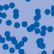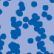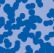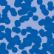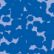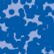## News

Prof. Dr. Karl-Theodor Sturm has been elected into the Academia Europaea. (28.06.2022)

Florian Schweiger erhielt den Hausdorff-Gedächtnispreis 2021 der Fachgruppe Mathematik für die beste Dissertation. Er fertigte die Dissertation unter der Betreuung von Prof. Stefan Müller an. Unter anderen wurde Vanessa Ryborz mit einem Preis der Bonner Mathematischen Gesellschaft für ihre von Prof. Sergio Conti betreute Bachelorarbeit ausgezeichnet. (18.01.2022)

Prof. Dr. Sergio Albeverio has been elected into the Academia Europaea and the Accademia Nazionale dei Lincei (more; 02.12.2021).

Der SFB 1060 Die Mathematik der emergenten Effekte hat eine dritte Förderperiode erhalten. (26.11.20)

Prof. Dr. Andreas Eberle erhält den diesjährigen Lehrpreis der Universität Bonn. (22.07.2020)

Contact

Managing Director: Prof. Dr. Massimiliano Gubinelli